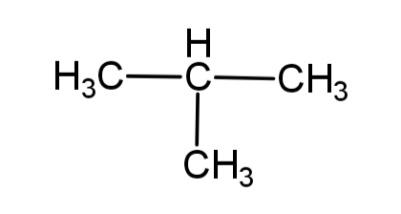Courses
Courses for Kids
Free study material
Offline Centres
MoreLast updated date: 25th Nov 2023
Total views: 278.1k
Views today: 5.78k

#a. Write the IUPAC name of this compoundb. Write the molecular formulac. Write the structural formula of its isomerd. Identify the type of isomerism in the above.Verified
278.1k+ views
Hint: IUPAC has made certain rules regarding the naming of organic compounds. The molecular formula of a compound tells us the total number of atoms present in their respective quantities. Isomers are compounds with the same molecular formula but different structures. There are various types of isomerism like, chain, stereo, geometric etc.

a. IUPAC has set norms regarding the naming of organic compounds. According to some of the rules:
-the longest hydrocarbon chain is selected.
-then according to the number of carbon atoms it is named like, methane, ethane, propane, etc.
-the closest carbon from the substituted or functional group is referred to as carbon – 1.
-the groups and the hydrocarbon chain are named in alphabetical order.
In the given compound there are 3 carbons in the longest chain and to the 2nd carbon is the methyl group attached, so this compound will be called 2 – methylpropane.
So, IUPAC name of the compound is 2 – methyl propane.
b. The molecular formula of any compound represents the total number of atoms present in the compound. The given compound is a hydrocarbon so it has C and H, the total number of carbons are 4 and the total number of hydrogen atoms are 10, so the molecular formula will be ${{C}_{4}}{{H}_{10}}$.
Hence, the molecular formula of the compound is ${{C}_{4}}{{H}_{10}}$.
c. Isomers of any compound have different arrangements of atoms. As 2 – methyl propane is a 3 carbon hydrocarbon, it can have 1 isomer that will be straight chain and it is written as:
$C{{H}_{3}}-C{{H}_{2}}-C{{H}_{2}}-C{{H}_{3}}$. This is the isomer of 2 – methyl propane.
d. The isomer consists of the change in the hydrocarbon chain position from branched to straight chain, so the type of isomerism is called chain isomerism.
Hence, the IUPAC name is 2 – methyl propane, with molecular formula ${{C}_{4}}{{H}_{10}}$and the isomerism as chain isomerism.

Note:
When isomers consist of arranging the functional groups then it is called functional isomerism, when the geometry is changed then it is called geometric isomerism. The molecular formula does not tell us the position of the hydrogen and the carbon atoms, the condensed structural formula can tell about the position of the atoms.